NBA英雄

# [流言板]预测下明年的表现吧！西蒙斯目前生涯4年场均15.9+8.1+7.7

252回复/ 0126201 浏览[流言板]预测下明年的表现吧！西蒙斯目前生涯4年场均15.9+8.1+7.7虎扑篮球资讯 发表在篮球资讯 https://bbs.hupu.com/502

15+9+8

15+9+8

17+8+8

17+8+8

15+9+8

15+9+8“神射手”

[图片]

[图片]

[图片]

[图片]

[图片]

[图片]

[图片]

[图片]

[图片]

“神射手”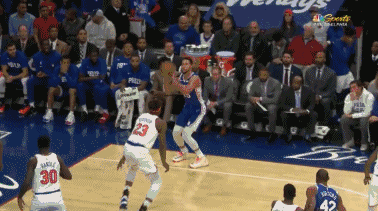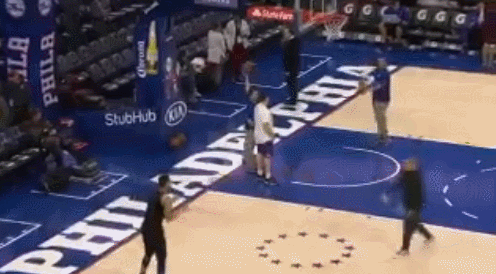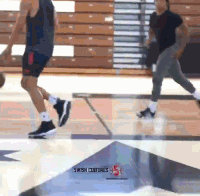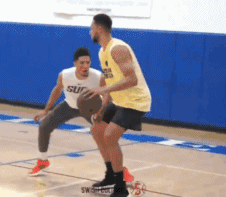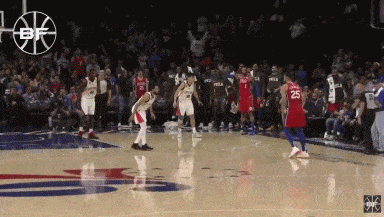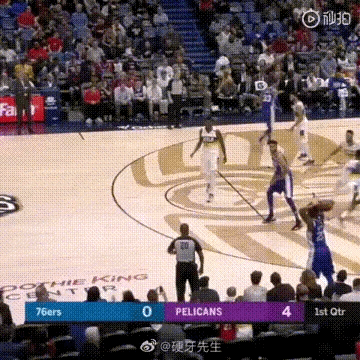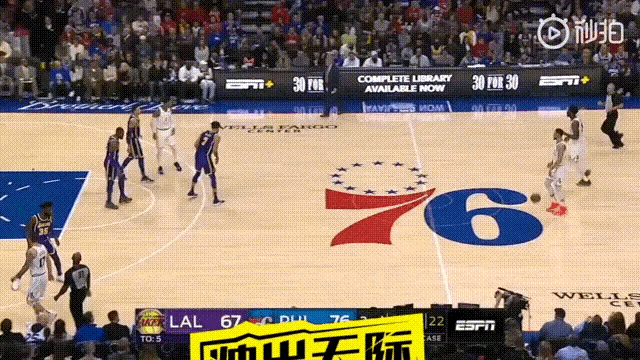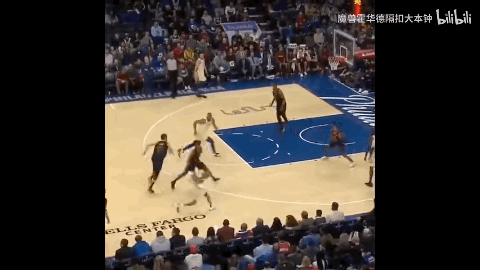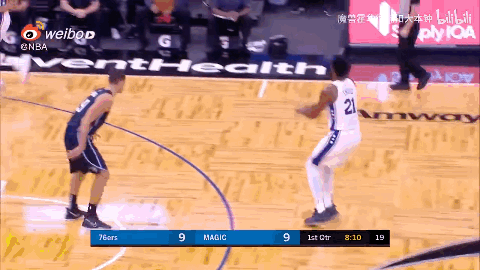15+9+8

15+9+8

“神射手”“神射手”“神射手”

[图片]

[图片]

[图片]

[图片]

[图片]

[图片]

[图片]

[图片]

[图片]

“神射手”[图片]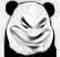15+9+8

15+9+8

17+8+8

17+8+8

18+8+918+8+915+10+6

15+10+613+7+6

13+7+6

15+9+8

15+9+828+7+7

28+7+7

18+8+818+8+815+9+8

15+9+8

“神射手”“神射手”18+8+8

18+8+828+7+7

28+7+7Re：[流言板]预测下明年的表现吧！西蒙斯目前生涯4年场均15.9+8.1+7.7